Journal of Behavioral Data Science, 2022, 2 (2), 73–98.
DOI: https://doi.org/10.35566/jbds/v2n2/suzuki

## Relative Predictive Performance of Treatments of Ordinal Outcome Variables across Machine Learning Algorithms and Class Distributions

University of North Carolina at Chapel Hill, Chapel Hill, NC, USA
hsuzuki@unc.edu
Abstract. Ordinal variables, such as those measured on a five-point Likert scale, are ubiquitous in the behavioral sciences. However, machine learning methods for modeling ordinal outcome variables (i.e., ordinal classification) are not as well-developed or widely utilized, compared to classification and regression methods for modeling nominal and continuous outcomes, respectively. Consequently, ordinal outcomes are often treated “naively” as nominal or continuous outcomes in practice. This study builds upon previous literature that has examined the predictive performance of such naïve approaches of treating ordinal outcome variables compared to ordinal classification methods in machine learning. We conducted a Monte Carlo simulation study to systematically assess the relative predictive performance of an ordinal classification approach proposed by Frank and Hall (2001) against naïve approaches according to two key factors that have received limited attention in previous literature: (1) the machine learning algorithm being used to implement the approaches and (2) the class distribution of the ordinal outcome variable. The consideration of these important, practical factors expands our knowledge on the consequences of naïve treatments of ordinal outcomes, which are shown in this study to vary substantially according to these factors. Given the ubiquity of ordinal measures coupled with the growing presence of machine learning applications in the behavioral sciences, these are important considerations for building high-performing predictive models in the field.

Keywords: Ordinal classification · Machine learning · Predictive performance · Class imbalance · Measurement scale

### 1 Introduction

In supervised learning, ordinal classification or equivalently ordinal regression, refers to a classification task where classes of the categorical outcome variable have an inherent ordering. This distinguishes it from nominal multi-class classification, where the classes are unordered. Ordinal classification is also distinct from regression where the outcome variable is continuous, because the numeric labels of the ordinal classes do not indicate equal spacing between adjacent classes. Ordinal measures are ubiquitous in a variety of disciplines, including the behavioral sciences. For example, human response data are often captured in Likert-type scales, such as those with response levels ranging from strongly disagree to strongly agree or poor to excellent.

Although not as well-developed and well-studied compared to nominal classification and regression (Ben-David, Sterling, & Tran2009Gutierrez, Perez-Ortiz, Sanchez-Monedero, Fernandez-Navarro, & Hervas-Martinez2016), machine learning methods for ordinal classification have been developed by many researchers. Some of these methods are modified versions of specific algorithms developed to handle ordinal outcome variables. This has been particularly popular with support vector machines (Chu & Keerthi2007Crammer & Singer2005Gu, Sheng, Tay, Romano, & Li2015Herbrich, Graepel, & Obermayer2000) and neutral networks (Cheng, Wang, & Pollastri2008Deng, Zheng, Lian, Chen, & Wang2010Fernandez-Navarro, Riccardi, & Carloni2014). Rather than modifying specific algorithms, researchers have also developed ordinal classification methods that can be implemented with multiple algorithms (Cardoso & da Costa2007Frank & Hall2001Lin & Li2012). For example, Frank and Hall (2001) proposed an approach to decompose an ordinal classification task into multiple binary classification tasks while retaining the ordinal information among classes, where any algorithm can be used as the base binary classifier, making it an algorithm-independent approach.

However, in practice, without strong familiarity with ordinal classification methods, machine learning researchers and practitioners may choose to implement a more “naïve” and easier approach to modeling ordinal outcome variables (Gutierrez et al.2016). This involves casting the ordinal outcome variable as a nominal variable, in which case the task at hand reduces to nominal multi-class classification. Similarly, the ordinal outcome variable may be cast as a continuous variable, in which case the task at hand becomes regression. To obtain integer-valued predictions in this case, some form of post-processing of the real-valued predictions, such as rounding, may be required (Kramer, Widmer, Pfahringer, & de Groeve2000). We refer to these naïve treatments of ordinal outcome variables as naïve classification and naïve regression, respectively.

Despite the developments in the ordinal classification literature, there are several possible reasons why researchers and practitioners may choose to implement a naïve approach. Bürkner and Vuorre (2019) discuss how it is common practice to treat ordinal measures as if continuous in the context of traditional statistical methods (e.g., t-tests, ANOVA, ordinary least squares regression). They provide several reasons for this, including hesitation due to perceived complexity in implementation or interpretation of ordinal approaches, difficulty in deciding which ordinal model to choose, or skepticism from journal editors and reviewers for using a “non-standard” approach (Bürkner & Vuorre2019). Although their discussion was in the context of traditional statistical methods, these reasons conceivably apply to machine learning contexts as well. Other factors may also include unfamiliarity or unavailability of accessible software to readily implement ordinal classification methods.

Given that naïve treatments of ordinal outcome variables are not uncommon, it is important to understand the consequences of implementing such naïve approaches. In the statistical literature, the consequences of treating ordinal measures as if continuous, rather than nominal, has specifically received attention. Liddell and Kruschke (2018) found this practice to be particularly common in psychological research, after surveying articles published in several highly ranked psychology journals. Motivated by this finding, the authors showed how systematic errors in analyses can arise from treating ordinal measures as continuous, including inflated Type I and Type II errors and misleading effect size estimates, and they suggest using models that allow for a proper treatment of ordinal variables (Liddell & Kruschke2018) . In the machine learning literature, the analogous investigation of the consequences of using naïve approaches are perhaps studies that compare ordinal classification methods against naïve classification or naïve regression in terms of predictive performance (Ben-David et al.2009Cardoso & da Costa2007Chu & Keerthi2007Frank & Hall2001Herbrich et al.2000Kramer et al.2000). In such studies, the naïve approaches are typically, although not always, shown to have lower predictive performance compared to an ordinal classification method, given that naïve approaches give a less than ideal treatment of ordinal variables by discarding the ordinal information (naïve classification) or assuming equal spacing between adjacent classes (naïve regression).

The present study builds upon this literature by investigating how ordinal classification methods perform relative to naïve classification and naïve regression according to key factors, including the machine learning algorithm being used to implement the approaches, the number of classes, and the degree of class imbalance present in the ordinal outcome variable. We do so by conducting a Monte Carlo simulation study to systematically evaluate predictive performance across different treatments of ordinal outcome variables, each implemented with multiple machine learning algorithms and crossing different levels of class imbalance with different numbers of classes in the ordinal outcome variable. This investigation is related to, but extends in important ways, previous studies in this line of research. For example, Gutierrez et al. (2016) examined the performance of naïve approaches and various ordinal classification methods on several datasets with varying numbers of ordinal classes. However, naïve approaches were only implemented using support vector machines, and varying degrees of class imbalance in the ordinal outcome variable were not varied or examined systematically with the number of classes. Cardoso and da Costa (2007) examined the performance of their data reduction method for ordinal classification compared to naïve approaches, implemented with support vector machines and neural networks. However, their study also did not systematically examine the impact of class distributions in the ordinal outcome variable. Similarly, Ben-David et al. (2009) used logistic regression and support vector machines to compare the performance of ordinal classification methods based on these algorithms compared to naïve classification. However, they did not examine class distributions or the performance of naïve regression using these same algorithms.

Researchers and practitioners routinely work with multiple algorithms in a given task, and many ordinal variables in real data tend to exhibit class imbalance (Baccianella, Esuli, & Sebastiani2009). As such, investigating how predictive performance compares across different treatments of the ordinal outcome variables in light of these practical considerations would expand our knowledge on the consequences of resorting to a naïve approach under various conditions and encourage more informed choices around the treatment of ordinal variables in practice.

### 2 Methods

We compared the predictive performance of three treatments of ordinal outcome variables: as a nominal variable in naïve classification, as a continuous variable in naïve regression, and as an ordinal variable in an ordinal classification method. For the ordinal classification method, we chose to employ the algorithm-independent approach proposed by Frank and Hall (2001). We chose this approach because it can be implemented with multiple algorithms, which allows for more intuitive comparisons in the relative predictive performance of the ordinal classification method across algorithms. Moreover, the approach is simple and intuitive. Given the practical barriers to employing ordinal classification methods discussed above, it may be most useful to examine the relative performance of an ordinal classification method that practitioners are most realistically likely to implement and that does not require much heavy lifting from naïve approaches.

#### 2.1 Frank and Hall (2001) Approach

Given an ordinal classification task with $k$ ordinal classes, the Frank and Hall (2001) approach (“FH approach” hereafter) involves training $k-1$ binary classifiers on $k-1$ modified copies of the original dataset. The $j^{th}$ binary classifier is trained on a modified outcome variable that is a binary indicator for whether or not the original outcome is greater than the $j^{th}$ ordered class. The predictors remain unchanged. For example, with $k=3$ (class 1 $<$ class 2 $<$ class 3), two binary classifiers are trained, where the first classifier predicts whether or not the outcome is greater than class 1 (i.e., class 2 or 3), and the second classifier predicts whether or not the outcome is greater than class 2 (i.e., class 3). Using the predicted probabilities from the $k-1$ binary classifiers, the predicted probability that an observation belongs to each of the $k$ classes is obtained in the following manner:Once the $k$ predicted probabilities are calculated per observation, an observation is assigned to the class with the greatest predicted probability.

In their study, Frank and Hall (2001) demonstrated this approach on many benchmark regression datasets by discretizing the continuous outcomes into $k$ balanced ordinal classes. They used C4.5 as the base algorithm and evaluated predictive performance with accuracy (1 minus misclassification rate). Using $k$ = 3, 5, and 10, they found higher accuracy associated with the FH approach across most datasets, compared to naïve classification (i.e., C4.5 treating the outcome as a nominal variable). They also found this performance gap between the FH approach and naïve classification to increase with $k$. The present study extends these evaluations by implementing the FH approach with more algorithms besides C4.5, considering imbalanced ordinal classes, comparing the predictive performance of the FH approach against naïve regression in addition to naïve classification, and considering additional performance metrics besides the misclassification rate.

#### 2.2 Algorithms Used

We implemented each of the three approaches (naïve classification, naïve regression, and FH approach) with two machine learning algorithms: classification and regression trees (CART; Breiman, Friedman, Olshen, & Stone2017) and random forests (Breiman2001). These tree-based algorithms have become increasingly popular among researchers in many disciplines including psychology, due to their desirable qualities such as ease of application and interpretability (Strobl, Malley, & Tutz2009). Despite their popularity, these algorithms’ performance across naïve and ordinal classification methods has not been examined as extensively compared to other algorithms, such as support vector machines and neural networks.

#### 2.3 Performance Metrics

Although the misclassification rate is a popular performance metric for classification tasks, it is not ideal for ordinal classification tasks because it gives equal penalty to all types of misclassifications (Gaudette & Japkowicz2009). For example, misclassifying a strongly disagree response as disagree is not as detrimental of an error as misclassifying a strongly disagree response as strongly agree. As such, we used two additional performance metrics besides the misclassification rate to evaluate the approaches: mean absolute error (MAE) and Spearman’s correlation coefficient. With observed numeric class labels $y$ and predicted numeric class labels $\hat {y}$, mean absolute error is calculated as $N^{-1}\sum _{i=1}^{N}|y_{i}-\hat {y}_{i}|$. Spearman’s correlation coefficient is calculated as $1-{6\sum _{i=1}^{N}{D_{i}}^{2}}/[{N(N^{2}-1)}]$, where $D_{i}$ refers to the difference between the rank order of $y_{i}$ and that of $\hat {y}_{i}$. These measures better account for the severity of error based on the ordinal information by using the numeric labels of the ordinal classes. These metrics have also been used in several studies that have examined the performance of ordinal classification approaches (Cardoso & da Costa2007Gutierrez et al.2016Kramer et al.2000).

In addition to evaluating overall predictive performance of each approach, we also evaluated the predictive performance of each approach at the class-level. For class-level performance, we examined the F1 score per class. The F1 score captures a balance of precision (proportion of true positives out of predicted positives) and recall (proportion of true positives out of actual positives) and can be calculated as ${2 {*Precision*Recall}}/({ {Precision}+ {Recall}})$. A “positive” case in this context refers to an observation belonging to a given class, and a “negative” case refers to an observation belonging to all other classes.

#### 2.4 Simulation Design and Analysis Plan

To investigate our research question, we conducted Monte Carlo simulations using the R statistical software (R Core Team2022). There were two simulation factors: the number of ordinal classes in the outcome variable ($k$ = 3, 5, 7) and the degree of class imbalance present in the ordinal outcome variable (we termed them balanced, slightly imbalanced, imbalanced class distributions). This gave a total of nine conditions, and each condition contained 500 replications. For each replication, we simulated a dataset of 2,000 observations using the mlbench.friedman1 function from the mlbench package (Leisch & Dimitriadou2021). This generates a benchmark regression dataset with ten predictors ($x_{1}$ through $x_{10}$) from a uniform distribution bounded by 0 and 1 and an error term from the standard normal distribution. Then, a subset of these predictors is used to generate a continuous outcome variable $y$, wherewhich we discretized into $k$ balanced, slightly imbalanced, or imbalanced classes, depending on the condition. For the balanced condition, each class contained ${1}/{k}$ of the observations. For the slightly imbalanced condition, the $j^{th}$ class contained $1/k+ [j-0.5 (k+1 )]/k^{2}$ of the observations. For the imbalanced condition, the $j^{th}$ class containedof the observations. To better visualize these proportions, Figure 1 presents the class distributions that result from the above rules for $k$ = 3, 5, and 7, along with measures of skewness and kurtosis for each distribution. Note that these rules can be used to generate imbalanced and slightly imbalanced class distributions for any value of $k$.(a) Slightly imbalanced condition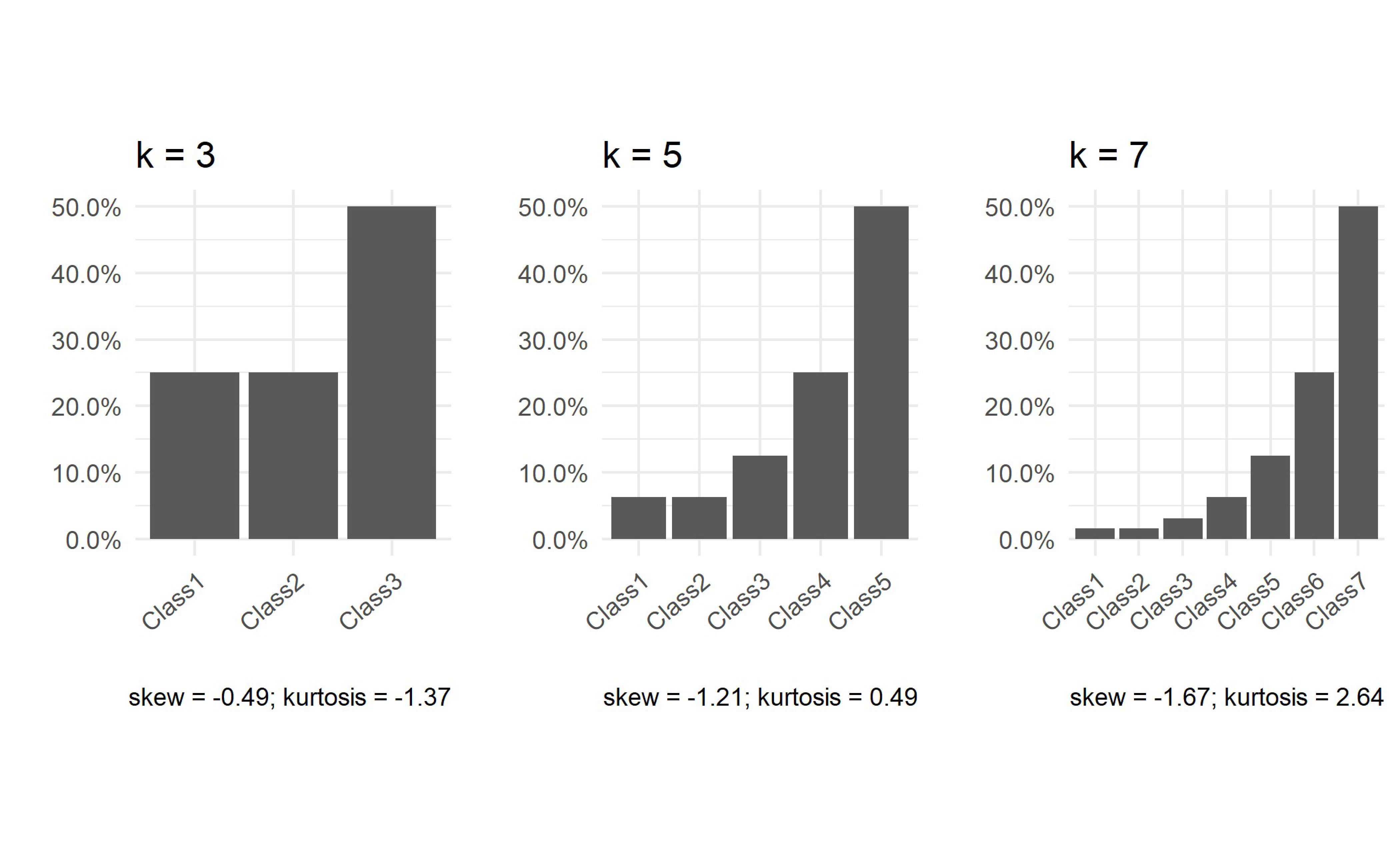(b) Imbalanced condition

Figure 1: Class distributions for the slightly imbalanced (Panel a) and imbalanced (Panel b) conditions

In each dataset, we implemented the two algorithms, CART and random forest, using naive classification, naïve regression, and the FH approach, for a total of six models per replication. Models were built using the caret package (Kuhn2022), by calling the rpart (Therneau & Atkinson2022) and rf (Liaw & Wiener2002) methods for CART and random forest, respectively. We trained these models and tuned hyperparameters with five-fold cross validation using 50% (N = 1,000) of the dataset and evaluated their predictive performance on the remaining 50% (N = 1,000) of the dataset. Note that for naïve regression, real-valued predictions were rounded to the nearest class. For CART, we tuned the complexity parameter, which is the factor by which any additional split attempted in the tree must decrease the prediction error. For random forests, we tuned the number of predictors that are randomly selected as split candidates at each split in a tree. For each of the six models per replication, we recorded the misclassification rate, MAE, and Spearman’s correlation as measures of overall predictive performance, and we recorded the F1 score for each of $k$ classes as a measure of class-level performance.

Within each algorithm in each condition, we examined the mean difference in overall predictive performance (for each of the three overall performance metrics) between the FH approach and naïve classification and between the FH approach and naïve regression. We first qualitatively examined the patterns by plotting the distributions of the performance metrics via boxplots. We then conducted paired samples t-tests and calculated effect sizes of the paired differences in performance across the approaches for each algorithm and condition to quantitatively examine patterns. We similarly calculated effect sizes for the paired differences in class-level performance between the FH approach and naïve approaches for each algorithm and condition.

In addition to the simulation study, we demonstrate the practical implementation of the FH approach in Appendix A, which contains step-by-step R code to carry out the FH approach using CART and random forests (but can easily be adapted to use any other algorithm) with an empirical example, as well as the resulting overall and class-level performance of the FH and naïve approaches on this empirical dataset.

### 3 Results

The overall performance results using Spearman’s correlation and MAE led to largely the same conclusions. For brevity, we focus our discussion on the results using Spearman’s correlation. There were a few differences in results when evaluating overall performance using the misclassification rate, which we summarize towards the end of this section. Results based on MAE and the misclassification rate can be found in Appendix B.

#### 3.1 CART Implementation

The CART implementation produced results that extend Frank and Hall’s (2001) findings well. Figure 2 presents the distribution of the six models’ predictive performance in terms of Spearman’s correlation across the 500 replications. Each square subplot represents a condition defined by the number of classes $k$ and the degree of class imbalance. Qualitatively, Figure 2 shows that with CART, the FH approach outperformed (i.e., higher Spearman’s correlations) both naïve classification and naïve regression across all nine conditions, including the imbalanced and slightly imbalanced conditions. Further, the performance gaps between the FH and naïve approaches generally appear to grow with a larger $k$ within each level of class imbalance.

To examine these observations quantitatively, we conducted paired samples t-tests within each set of 500 replications (i.e., within each of the nine conditions) per algorithm to compare the overall performance of the FH approach to each of the naive approaches. The mean paired difference (and standard deviation of the difference) in Spearman’s correlation for each algorithm in each condition are presented in Table 1, as well as the effect sizes in Cohen’s d for those differences (Cohen1988). Differences were calculated as Spearman’s correlation of the FH approach minus Spearman’s correlation of the naïve approaches. Thus, a positive mean difference and effect size indicate the FH approach performing better, and a negative mean difference and effect size indicate the FH approach performing worse compared to the naïve approaches. Effect sizes are also visualized in Figure 3.

Table 1: Mean paired differences (and standard deviations) in overall predictive performance in terms of Spearman’s correlation between the FH approach and naive approaches for each algorithm in each simulation condition; effect sizes of the paired differences
 k Degree of class balance CART Naïve Classification Naïve Regression Balanced .015 (.023)*; .674 .035 (.025)*; 1.388 3 Slightly Imbalanced .021 (.024)*; .850 .037 (.027)*; 1.384 Imbalanced .023 (.028)*; .797 .038 (.027)*; 1.425 Balanced .047 (.023)*; 2.079 .061 (.022)*; 2.788 5 Slightly Imbalanced .049 (.022)*; 2.209 .048 (.019)*; 2.534 Imbalanced .033 (.028)*; 1.170 .058 (.025)*; 2.267 Balanced .066 (.026)*; 2.527 .059 (.015)*; 3.836 7 Slightly Imbalanced .069 (.026)*; 2.681 .063 (.018)*; 3.547 Imbalanced .036 (.029)*; 1.212 .065 (.027)*; 2.386 Random Forest Naïve Classification Naïve Regression Balanced -.003 (.010)*; -.315 -.001 (.012); -.068 3 Slightly Imbalanced -.002 (.010)*; -.179 .003 (.011)*; .297 Imbalanced .002 (.011)*; .218 .006 (.014)*; .426 Balanced -.001 (.008)*; -.167 -.027 (.008)*; -3.234 5 Slightly Imbalanced .000 (.008); -.014 -.023 (.008)*; -2.737 Imbalanced -.002 (.013); -.127 -.018 (.015)*; -1.211 Balanced -.001 (.008); -.082 -.038 (.007)*; -5.149 7 Slightly Imbalanced .001 (.009); .130 -.036 (.008)*; -4.343 Imbalanced -.003 (.014)*; -.178 -.024 (.017)*; -1.471

Note. *$p<\frac {.05}{36}$ (Bonferroni-corrected); bold indicates moderate or large effect size ($|d|>0.5$). Positive mean differences and effect sizes indicate the FH approach performing better than the naïve approach.

Table 1 shows that for the CART implementation, as qualitatively observed in Figure 2, the FH approach had significantly better performance than both naïve approaches. This is indicated by the mean differences in predictive performance being positive and significant ($p<\frac {.05}{36}$; Bonferroni-corrected alpha level for multiple testing) for both naïve classification and naïve regression in all nine conditions. Further, effect sizes were all at least in the moderate range ($d>.5$), and effect sizes grew more positive with a larger $k$, as illustrated in Figure 3. Overall, we observed comparable effect sizes between balanced and slightly imbalanced classes, while imbalanced classes tended to have smaller effect sizes. These results indicate that with CART, the FH approach resulted in improved predictive performance over both naïve approaches, and that this performance boost was most prevalent in conditions with more classes and more class balance.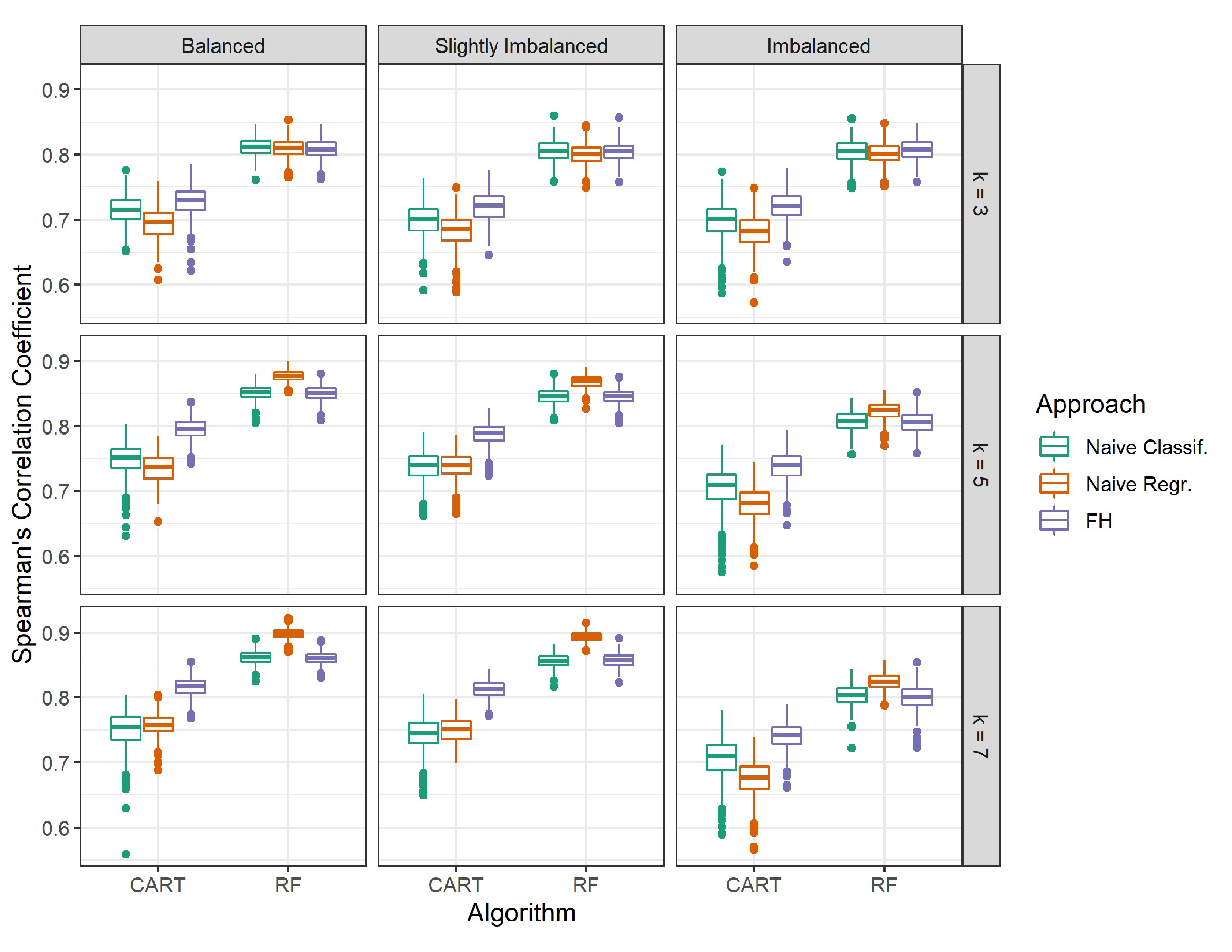Figure 2: Distribution of overall predictive performance (Spearman’s correlation) of the six models across replications in each simulation condition

Figure 4(a) plots the effect sizes of the paired differences in class-level F1 scores between the FH and naïve approaches for the CART implementation in each condition. It is interesting to note that in all conditions, not all class-level effect sizes were positive and at least moderate ($d>.5$), meaning class-level performance was only higher for the FH approach in some classes, even though overall performance was higher. For example, there appeared to be a pattern in nearly all conditions, where there was a negligible difference in performance between the FH approach and naïve classification for the “end” classes (i.e., classes 1 and $k$), as indicated by near-zero effect sizes, but the FH approach outperformed naïve classification for the “middle” classes (i.e., classes $2,\ldots ,k-1$), as indicated by positive effect sizes. There were no such apparent patterns for naïve regression, but there were similarly some classes where the FH approach outperformed naïve regression and other classes where there were negligible differences. These class-level effect sizes indicate that the improvement in overall predictive performance associated with the FH approach in a given condition did not necessarily come from a uniform improvement in performance across all classes, but only in some.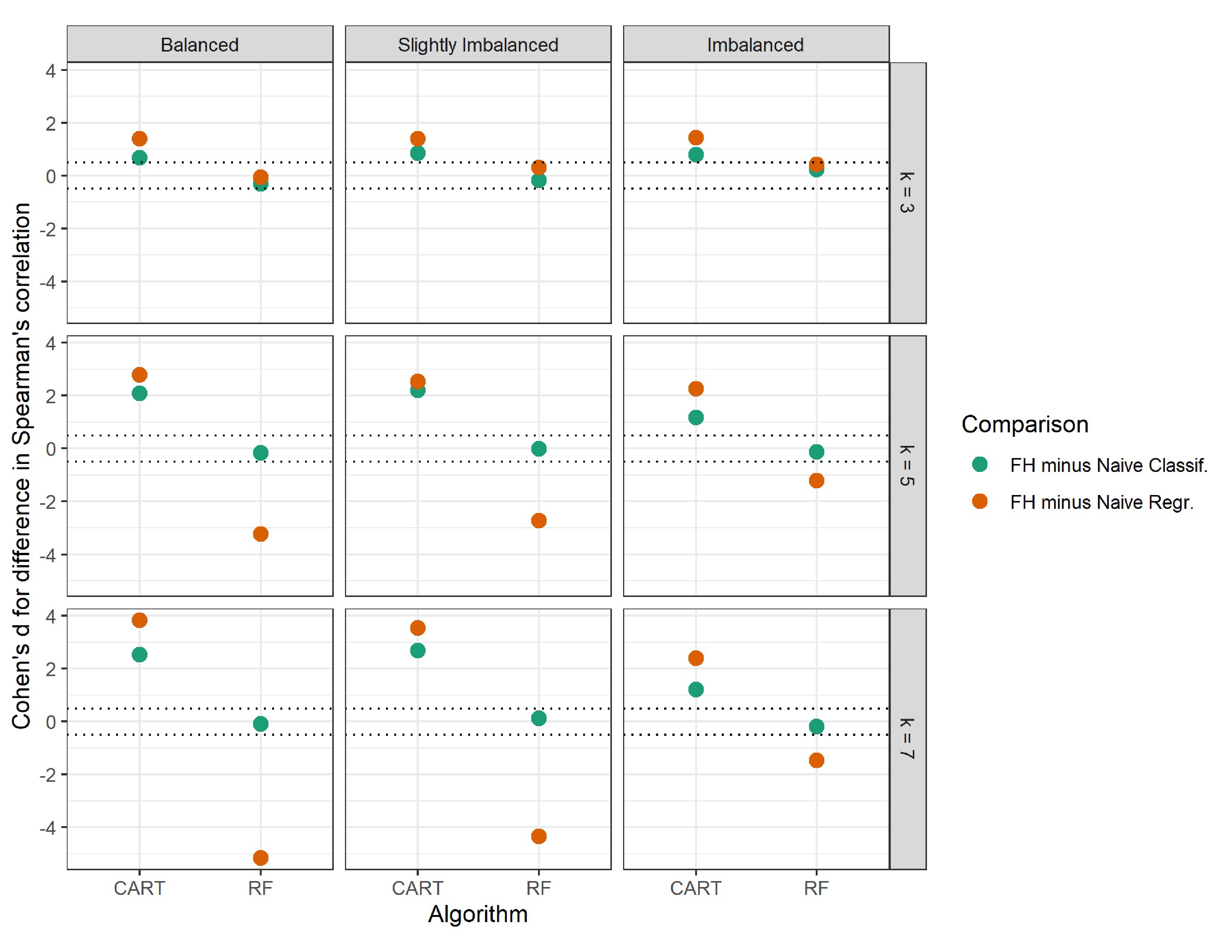Figure 3: Effect sizes for the paired difference in overall predictive performance (Spearman’s correlation) between the FH approach and naïve approaches for each algorithm in each simulation condition. Dashed lines appear at $|d|=0.5$.

#### 3.2 Random Forest Implementation

The random forest implementation produced less intuitive results compared to the CART implementation. First, Figure 2 shows that when comparing the FH approach to naïve classification, there were virtually no differences in overall predictive performance between these two approaches in all conditions, meaning that the FH approach did not perform any better than naïve classification. Second, Figure 2 shows that when comparing the FH approach to naïve regression, in conditions with $k=3$, these two approaches also performed similarly, meaning the FH approach did not perform any better than naïve regression, either. However, in conditions with a larger $k$, naïve regression outperformed the FH approach. The mean differences and effect sizes in Table 1 corroborate these observations. While we did observe some significant mean differences ($p<\frac {.05}{36}$) in certain conditions for naïve classification, all effect sizes were small ($|d|<.5$). For naïve regression, we observed negative and significant mean differences with large effect sizes in conditions with $k=5$ and 7. Figure 3 also illustrates these patterns. The green (naïve classification) effect sizes hovered around zero in all conditions, indicating no difference in overall performance between the FH approach and naïve classification. The orange (naïve regression) effect sizes, under $k=3$, also hovered around zero, but with a larger $k$, they were negative. These effect sizes grew more negative with more class balance, indicating that naïve regression increasingly outperformed the FH approach with more class balance.

Figure 4(b) plots the effect sizes for the paired differences in class-level F1 scores between the FH approach and naïve approaches for the random forest implementation in each condition. For naïve classification, effect sizes generally hovered around zero in most conditions, indicating no difference in class-level performance between the FH approach and naïve classification. For naïve regression, there appeared to be a pattern in nearly all conditions where the “end” classes (i.e., classes 1 and $k$) had positive effect sizes, indicating that the FH approach outperformed naïve regression, but the “middle” classes (i.e., classes $2,\ldots ,k-1$) had negative effect sizes, indicating that the FH approach performed worse than naïve regression. These class-level effect sizes show that the relative class-level performance of these approaches can differ according to class and may not all be in the same direction as the overall relative performance.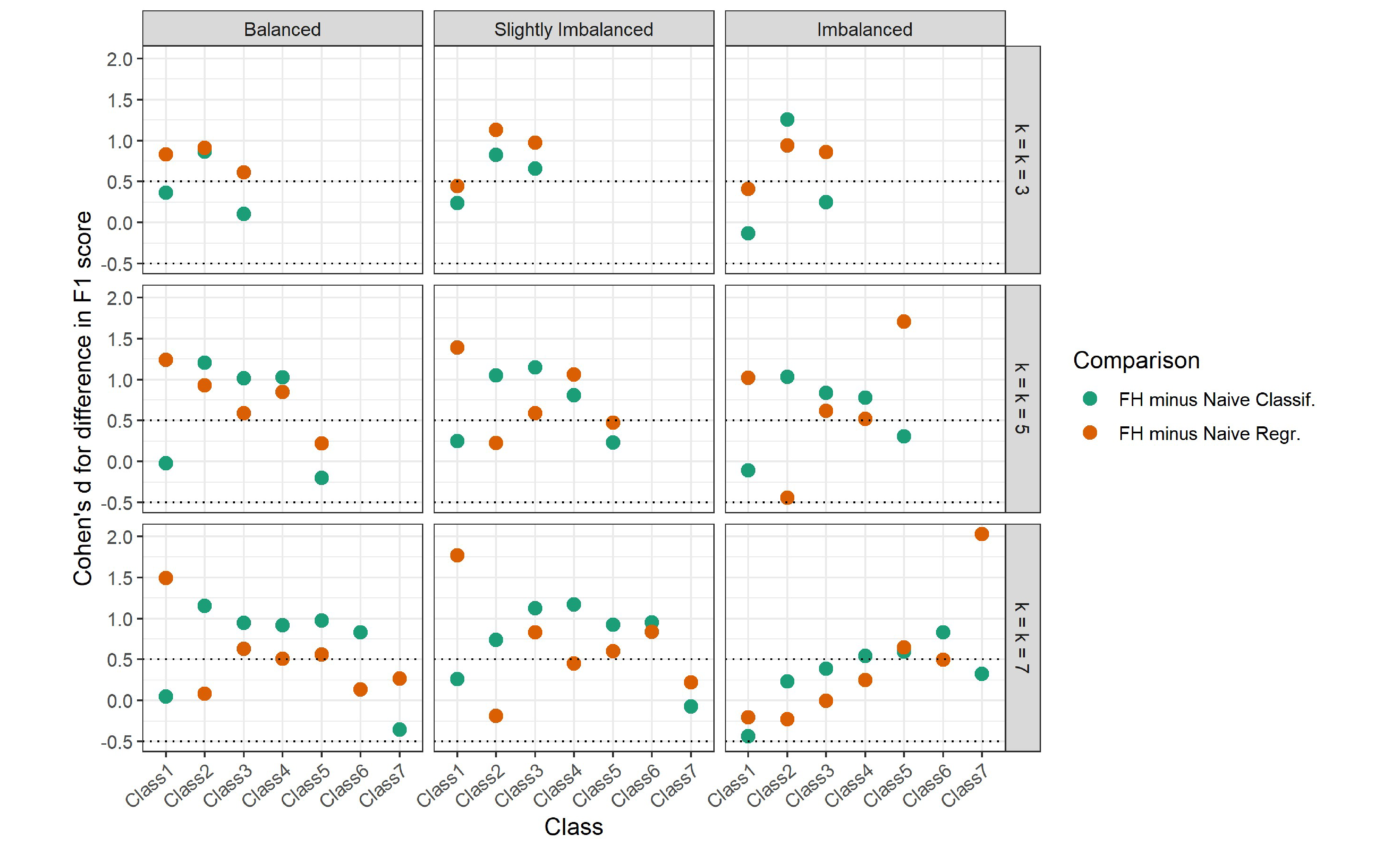(a) CART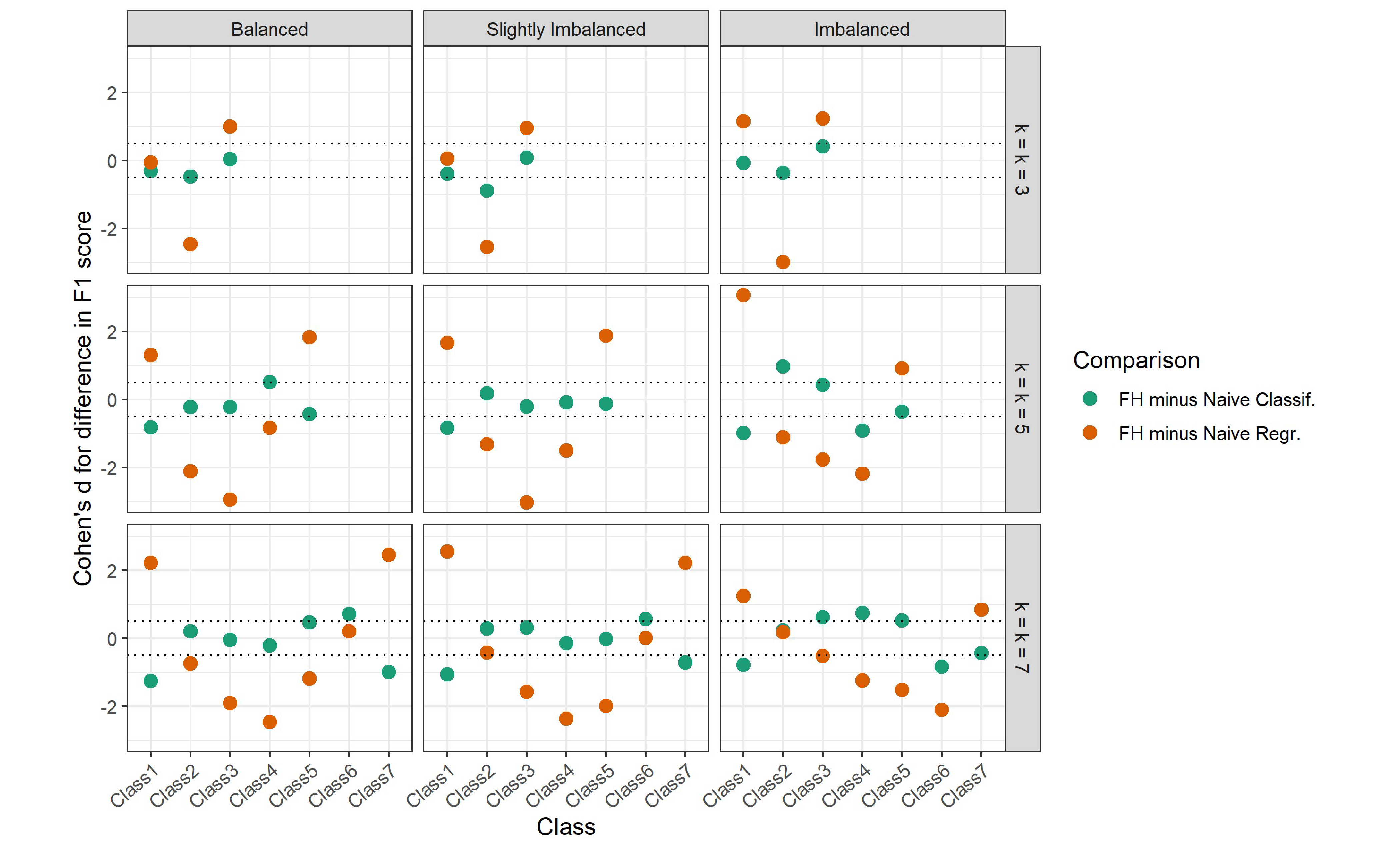(b) Random forest

Figure 4: Effect sizes for the paired difference in class-level predictive performance (F1 score) between the FH approach and naïve approaches for CART (Panel a) and random forests (Panel b) in each simulation condition. Dashed lines appear at $|d|=0.5$.

#### 3.3 Summary of Results

With the CART implementation, the FH approach had significantly better overall performance than both naïve classification and naïve regression across all nine conditions, including those with imbalanced and slightly imbalanced class distributions. The overall performance gap between the FH approach and naïve approaches grew with $k$. With the random forest implementation, the FH approach did not perform differently from naïve classification in any meaningful way across all nine conditions. However, the FH approach performed significantly worse compared to naïve regression in conditions with a larger $k$, and the performance gap increased with more class balance. In both CART and random forest implementations, we found that in a given condition, class-level performance was not uniformly better or worse for the FH approach across classes according to the overall performance results. For example, even when overall performance was substantially higher for the FH approach than a naïve approach in a given condition, this did not mean that the FH approach had accordingly higher class-level performance in each class for that condition.

Using the misclassification rate as the overall performance metric led to largely the same findings as Spearman’s correlation throughout the CART and random forest implementations. The exception was that in the random forest implementation, the FH approach had comparable overall performance to both naïve classification and naïve regression across all conditions, rather than naïve regression outperforming the FH approach in some conditions. Given that the misclassification rate is an unsuitable performance metric for ordinal classification tasks, we do not expand on these findings. However, this does reveal that different conclusions can be made from using different overall performance metrics, highlighting the importance of using a metric that is most suited to the task at hand.

### 4 Discussion

Ordinal measures are ubiquitous, but given limitations in familiarity or availability of machine learning methods for ordinal classification, they may not always be treated as an ordinal variable in practice. In this study, we aimed to expand our understanding of the impacts of treatments of ordinal outcome variables on predictive performance across various conditions. Specifically, we used Monte Carlo simulations to examine the relative predictive performance of an ordinal classification method, namely the FH approach, against naïve classification and naïve regression according to the machine learning algorithm being implemented, the number of classes in the ordinal outcome variable, and the degree of class imbalance in the ordinal outcome variable.

Our results differed substantially across algorithms. With the CART implementation, results aligned well with and extended Frank and Hall’s (2001) findings. The FH approach was associated with a higher overall predictive performance compared to both naïve classification and naïve regression, and this pattern held across all conditions, even in the presence of class imbalance. The overall performance gap increased with the number of classes and was largest among balanced classes, indicating that the benefit of treating the outcome as an ordinal variable by implementing the FH approach is greatest when there are many, balanced classes.

On the other hand, we found some divergent results with the random forest implementation. The FH approach had comparable overall performance to naive classification in all conditions, and the FH approach performed worse than naive regression in conditions with more classes and more class balance. One possible explanation for this could be tied to the fact that random forests are ensemble learners that, in general, tend to have better predictive performance than weak learners like CART and C4.5. Thus, it is possible that the naïve approaches implemented with random forests already provided decent predictive performance that there may not have been as much to be gained from the implementation of the FH approach. Further, with a larger number of classes, the ordinal outcome variable might become better approximated as a continuous outcome, and perhaps that is why we observed naïve regression to perform particularly well in those conditions. In a similar study that examined the performance of various algorithm-independent ordinal classification methods, Hühn and Hüllermeier (2008) theorized that algorithms with more complex and flexible decision boundaries benefit less from incorporating the ordinal information among the classes of the outcome variable. This is consistent with findings from our study, as the random forest implementation of the FH approach, treating the outcome as an ordinal variable, did not result in increased predictive performance over the naïve approaches, whereas the CART implementation did.

In sum, these findings illustrate that the relative predictive performance of the different treatments of ordinal outcome variables varies across algorithms and conditions. In other words, the gain in overall predictive performance from treating an ordinal outcome properly as an ordinal variable by implementing the FH approach can depend on the number of classes, the degree of class imbalance present, and the algorithm being used. There is not always an improvement in overall predictive performance associated with the FH approach, and sometimes, naïve approaches may perform better than the FH approach. As such, there is no one approach that is always best, suggesting a need for careful and deliberate choices in the treatment of ordinal outcomes to achieve optimal predictive performance in machine learning.

#### 4.1 Limitations and Future Directions

There are several limitations associated with this study. First, the ordinal outcome variable in our simulated datasets were not “real” ordinal data, as the outcome variable was originally a continuous variable which was discretized. As such, the ordinal class structure may have been artificially accentuated compared to what is conceivable in naturally occurring ordinal data (Hühn & Hüllermeier2008). We used such simulated outcomes in order to be able to systematically manipulate and examine the influence of the number of classes and the degree of class imbalance in the ordinal outcome variable, which was a main goal of the study. Second, our findings are limited to the two specific algorithms, CART and random forest, that we implemented in this study. We chose these two algorithms as they have not received as much attention in the ordinal classification literature. However, there are many other binary classifiers that can be implemented with the FH approach, as this is an algorithm-independent approach. Given the surprising results we found with the random forest implementation, it would be interesting to study whether the same holds for other related methods, such as boosted trees (Hastie, Tibshirani, & Friedman2009). Similarly, we only examined one ordinal classification method to compare against naïve classification and naïve regression. Future studies should examine how other algorithm-independent ordinal classification methods provide similar or divergent results. Another direction for future study is to examine different shapes of class distributions. In this study, we only examined three levels of class imbalance, and the skewness of the imbalanced and slightly imbalanced distributions were in the same direction (i.e., all upwards sloping, where the most frequent class was class $k$). It would thus be interesting to examine how results may change with different shapes (e.g., downwards sloping with class 1 being the most frequent class, or a random pattern where class frequencies do not increase or decrease uniformly with class labels) and how this may impact class-level performance. Lastly, while Spearman’s correlation and MAE provide more suitable overall performance metrics for ordinal classification tasks than the misclassification rate, they are not the only metrics available, nor are they free of flaws themselves. A major limitation of MAE and Spearman’s correlation as performance metrics of ordinal classification tasks is that they are influenced by the choice of the numeric label given to the ordinal classes (Cardoso & Sousa2011). Other measures have been suggested that are not influenced by the numeric label of ordinal classes (Cardoso & Sousa2011), but we have maintained the use of these metrics for this study for ease of computation and readers’ familiarity.

#### 4.2 Conclusions

This simulation study highlighted the variability in relative predictive performance of common treatments of ordinal outcome variables in machine learning according to key factors, including the algorithm being used to implement the approaches and the degree of class imbalance in the ordinal outcome. The consideration of these important, practical factors extends the previous literature on ordinal classification and provides further knowledge on the consequences of naïve treatments of ordinal outcomes, which are shown to vary substantially according to these factors. Given the ubiquity of ordinal measures in the behavioral sciences coupled with the growing use of machine learning in the behavioral sciences, these are important considerations for building high-performing models in the field.

### References

Baccianella, S., Esuli, A., & Sebastiani, F. (2009). Evaluation measures for ordinal regression. In 2009 ninth international conference on intelligent systems design and applications. IEEE. doi: https://doi.org/10.1109/isda.2009.230

Ben-David, A., Sterling, L., & Tran, T. (2009, apr). Adding monotonicity to learning algorithms may impair their accuracy. Expert Systems with Applications, 36(3), 6627–6634. doi: https://doi.org/10.1016/j.eswa.2008.08.021

Breiman, L. (2001). Random forests. Machine Learning, 45(1), 5–32. doi: https://doi.org/10.1023/a:1010933404324

Breiman, L., Friedman, J. H., Olshen, R. A., & Stone, C. J. (2017). Classification and regression trees. Routledge. doi: https://doi.org/10.1201/9781315139470

Bürkner, P.-C., & Vuorre, M. (2019, feb). Ordinal regression models in psychology: A tutorial. Advances in Methods and Practices in Psychological Science, 2(1), 77–101. doi: https://doi.org/10.1177/2515245918823199

Cardoso, J. S., & da Costa, J. F. P. (2007). Learning to classify ordinal data: The data replication method. Journal of Machine Learning Research, 8(50), 1393–1429. Retrieved from http://jmlr.org/papers/v8/cardoso07a.html

Cardoso, J. S., & Sousa, R. (2011, dec). Measuring the performance of ordinal classification. International Journal of Pattern Recognition and Artificial Intelligence, 25(08), 1173–1195. doi: https://doi.org/10.1142/s0218001411009093

Cheng, J., Wang, Z., & Pollastri, G. (2008, jun). A neural network approach to ordinal regression. In 2008 IEEE international joint conference on neural networks (IEEE world congress on computational intelligence). IEEE. doi: https://doi.org/10.1109/ijcnn.2008.4633963

Chu, W., & Keerthi, S. S. (2007, mar). Support vector ordinal regression. Neural Computation, 19(3), 792–815. doi: https://doi.org/10.1162/neco.2007.19.3.792

Cohen, J. (1988). Statistical power analysis for the behavioral sciences (2nd ed.). Routledge. doi: https://doi.org/10.4324/9780203771587

Crammer, K., & Singer, Y. (2005, jan). Online ranking by projecting. Neural Computation, 17(1), 145–175. doi: https://doi.org/10.1162/0899766052530848

Deng, W.-Y., Zheng, Q.-H., Lian, S., Chen, L., & Wang, X. (2010, dec). Ordinal extreme learning machine. Neurocomputing, 74(1-3), 447–456. doi: https://doi.org/10.1016/j.neucom.2010.08.022

Fernandez-Navarro, F., Riccardi, A., & Carloni, S. (2014, nov). Ordinal neural networks without iterative tuning. IEEE Transactions on Neural Networks and Learning Systems, 25(11), 2075–2085. doi: https://doi.org/10.1109/tnnls.2014.2304976

Frank, E., & Hall, M. (2001). A simple approach to ordinal classification. In Machine learning: ECML 2001 (pp. 145–156). Springer Berlin Heidelberg. doi: https://doi.org/10.1007/3-540-44795-4_13

Gaudette, L., & Japkowicz, N. (2009). Evaluation methods for ordinal classification. In Advances in artificial intelligence (pp. 207–210). Springer Berlin Heidelberg. doi: https://doi.org/10.1007/978-3-642-01818-3_25

Gu, B., Sheng, V. S., Tay, K. Y., Romano, W., & Li, S. (2015, jul). Incremental support vector learning for ordinal regression. IEEE Transactions on Neural Networks and Learning Systems, 26(7), 1403–1416. doi: https://doi.org/10.1109/tnnls.2014.2342533

Gutierrez, P. A., Perez-Ortiz, M., Sanchez-Monedero, J., Fernandez-Navarro, F., & Hervas-Martinez, C. (2016, jan). Ordinal regression methods: Survey and experimental study. IEEE Transactions on Knowledge and Data Engineering, 28(1), 127–146. doi: https://doi.org/10.1109/tkde.2015.2457911

Hastie, T., Tibshirani, R., & Friedman, J. (2009). The elements of statistical learning. Springer New York. doi: https://doi.org/10.1007/978-0-387-84858-7

Herbrich, R., Graepel, T., & Obermayer, K. (2000). Large margin rank boundaries for ordinal regression. In P. J. Bartlett, B. Schölkopf, D. Schuurmans, & A. J. Smola (Eds.), Advances in large margin classifiers (pp. 115–132). MIT Press.

Hühn, J. C., & Hüllermeier, E. (2008). Is an ordinal class structure useful in classifier learning? International Journal of Data Mining, Modelling and Management, 1(1), 45. doi: https://doi.org/10.1504/ijdmmm.2008.022537

Kramer, S., Widmer, G., Pfahringer, B., & de Groeve, M.  (2000). Prediction of ordinal classes using regression trees. In Lecture notes in computer science (pp. 426–434). Springer Berlin Heidelberg. doi: https://doi.org/10.1007/3-540-39963-1_45

Kuhn, M. (2022). caret: Classification and regression training [Computer software manual]. Retrieved from https://github.com/topepo/caret/ (R package version 6.0-93)

Leisch, F., & Dimitriadou, E. (2021). mlbench: Machine learning benchmark problems. Retrieved from https://cran.r-project.org/package=mlbench (R package version 2.1-3.)

Liaw, A., & Wiener, M. (2002). Classification and regression by randomForest. R News, 2(3), 18–22. Retrieved from http://CRAN.R-project.org/doc/Rnews/

Liddell, T. M., & Kruschke, J. K. (2018, nov). Analyzing ordinal data with metric models: What could possibly go wrong? Journal of Experimental Social Psychology, 79, 328–348. doi: https://doi.org/10.1016/j.jesp.2018.08.009

Lin, H.-T., & Li, L. (2012, may). Reduction from cost-sensitive ordinal ranking to weighted binary classification. Neural Computation, 24(5), 1329–1367. doi: https://doi.org/10.1162/neco_a_00265

R Core Team. (2022). R: a language and environment for statistical computing. Vienna, Austria: R Foundation for Statistical Computing. Retrieved from http://www.r-project.org

Strobl, C., Malley, J., & Tutz, G. (2009, dec). An introduction to recursive partitioning: Rationale, application, and characteristics of classification and regression trees, bagging, and random forests. Psychological Methods, 14(4), 323–348. doi: https://doi.org/10.1037/a0016973

Therneau, T., & Atkinson, B. (2022). rpart: Recursive partitioning and regression trees [Computer software manual]. Retrieved from https://CRAN.R-project.org/package=rpart (R package version 4.1.16)

### A Sample R Code

Below, we provide sample R code to demonstrate the implementation of the FH approach using CART and random forests on an applied dataset. The dataset (N = 1,014) contains predictors of maternal health risk among pregnant patients, including age, systolic and diastolic blood pressure, blood glucose levels, body temperature, and heart rate. The task is to predict maternal mortality risk level, an ordinal outcome variable with $k$ = 3 classes of low, mid, and high risk. These classes are distributed in the following manner: low risk (40.0%), mid risk (33.1%), and high risk (26.8%). The dataset is publicly available from the UCI Machine Learning Repository (https://archive.ics.uci.edu/ml/datasets/Maternal+Health+Risk+Data+Set). Note that the code can easily be adapted to implement the FH approach using other algorithms besides CART and random forests and on datasets with different values of $k$. The models are trained using the caret package, which streamlines the model training and hyperparameter tuning processes and provides a unified syntax for fitting different algorithms.

The dataset is saved in a data.frame object called data. The outcome variable is a column in data called RiskLevel.

First, we code the outcome variable as an ordinal variable. We save the class labels and the number of classes into respective objects to be referenced throughout the rest of the program.

data$RiskLevel = factor(data$RiskLevel,
levels = c("low risk",
"mid risk", "high risk"),
ordered = TRUE)
classes = levels(data$RiskLevel) k = length(classes) Next, we split the dataset into a training (50%) and test (50%) set. set.seed(12345) train = sample(seq_len(nrow(data)), size = floor(nrow(data)/2), replace = FALSE) dtrain = data[train, ] dtest = data[-train, ] To implement the FH approach, we generate$k$– 1 modified copies of the training set and save each one into a list called dtrain_modified. The$j^{th}$training set has a modified outcome variable that is a binary indicator for whether the original outcome is greater than the$j^{th}$class. The predictors remain unchanged. dtrain_modified = list() for (j in 1:(k-1)){ dt = dtrain dt$RiskLevel = as.factor(ifelse(dtrain$RiskLevel > classes[j], 1, 0)) dtrain_modified[[j]] = dt } We initialize two$N_{testset}$by ($k$– 1) matrices, one for each algorithm, to store the predicted probabilities from the$k$– 1 binary classifiers. probsCART = probsRF = matrix(ncol = k-1, nrow = nrow(dtest)) We are using the caret package to train the CART and random forest models. Below, we set up a control parameter for conducting 5-fold cross validation during training for hyperparameter tuning. library(caret) trnCntrl = trainControl(method =’cv’, number = 5) Below is the for-loop where we train the$k$– 1 binary classifiers per algorithm. In the$j^{th}$iteration (there are$k$– 1 iterations) of the loop, we train a CART model and a random forest model on the$j^{th}$modified training set. After training each model per iteration, we obtain predicted probabilities on the test set and save them into the$j^{th}$column of the matrix we initialized above. To use a different algorithm, simply change the method argument inside of the train function. for (j in 1:(k-1)){ # CART modCART_j = train(RiskLevel ~ ., data = dtrain_modified[[j]], method = ’rpart’, tuneLength = 10, trControl = trnCntrl) pred = predict(modCART_j$finalModel, dtest,
type = "prob")[,"1"]
probsCART[,j] = pred

# random forest
modRF_j = train(RiskLevel ~ .,
data = dtrain_modified[[j]],
method = ’rf’,
tuneLength = 5,
trControl = trnCntrl)
pred = predict(modRF_j$finalModel, dtest, type = "prob")[,"1"] probsRF[,j] = pred } Next, we combine the$k$– 1 predicted probabilities from the$k$– 1 binary classifiers to obtain predicted probabilities for each of the$k$classes. We initialize two$N_{testset}$by$k$matrices, one for each algorithm, to store these probabilities. probsCART_k = probsRF_k = data.frame(matrix(ncol = k, nrow = nrow(dtest))) colnames(probsCART_k) = colnames(probsRF_k) = classes The$k$– 1 predicted probabilities are combined according to the rules described in the Methods section of the study to obtain the$k$predicted probabilities. probsCART_k[,1] = 1 - probsCART[, 1] probsCART_k[,k] = probsCART[, (k-1)] probsRF_k[,1] = 1 - probsRF[, 1] probsRF_k[,k] = probsRF[, (k-1)] for (i in 2:(k-1)){ probsCART_k[,i] = probsCART[, (i-1)] - probsCART[, i] probsRF_k[,i] = probsRF[, (i-1)] - probsRF[, i] } Finally, each test set observation is assigned to the ordinal class with the largest predicted probability. We store the predicted class labels into a vector for each algorithm, which can be used to compute overall performance metrics, such as the mean absolute error and Spearman’s correlation. predclassCART = colnames(probsCART_k)[max.col(probsCART_k, ties.method = "random")] predclassCART = factor(predclassCART, levels = classes) predclassRF = colnames(probsRF_k)[max.col(probsRF_k, ties.method = "random")] predclassRF = factor(predclassRF, levels = classes) # Mean absolute error mean(abs(as.integer(predclassCART) - as.integer(dtest$RiskLevel)))
mean(abs(as.integer(predclassRF)
- as.integer(dtest$RiskLevel))) # Spearman cor(as.integer(predclassCART), as.integer(dtest$RiskLevel),
method = "spearman")
cor(as.integer(predclassRF), as.integer(dtest$RiskLevel), method = "spearman") Class-level performance can conveniently be obtained using the confusionMatrix function of the caret package. # class-level performance cmCART = confusionMatrix(predclassCART, dtest$RiskLevel)
cmRF = confusionMatrix(predclassRF, dtest$RiskLevel) cmCART$byClass
cmRF$byClass In the table below, we summarize the empirical performance results of the FH approach using CART and random forests on this applied dataset, along with the performance of the naïve classification and naïve regression approaches. Naïve classification and naïve regression can be implemented by simply converting data$RiskLevel into an unordered factor and into an integer, respectively. These results show that the FH approach generally outperformed both naïve approaches, although the improvement is minimal, especially for the random forest implementation.

 CART Performance metric FH Naïve classification Naïve regression Overall performance Spearman’s correlation .73 .67 .69 Mean absolute error .30 .35 .33 Misclassification rate .29 .32 .33 Class-level performance (F1) Low risk class .73 .70 .68 Mid risk class .57 .53 .58 High risk class .85 .83 .80 Random forest FH Naïve classification Naïve regression Overall performance Spearman’s correlation .80 .80 .78 Mean absolute error .20 .22 .24 Misclassification rate .19 .20 .23 Class-level performance (F1) Low risk class .82 .81 .76 Mid risk class .75 .71 .70 High risk class .90 .89 .88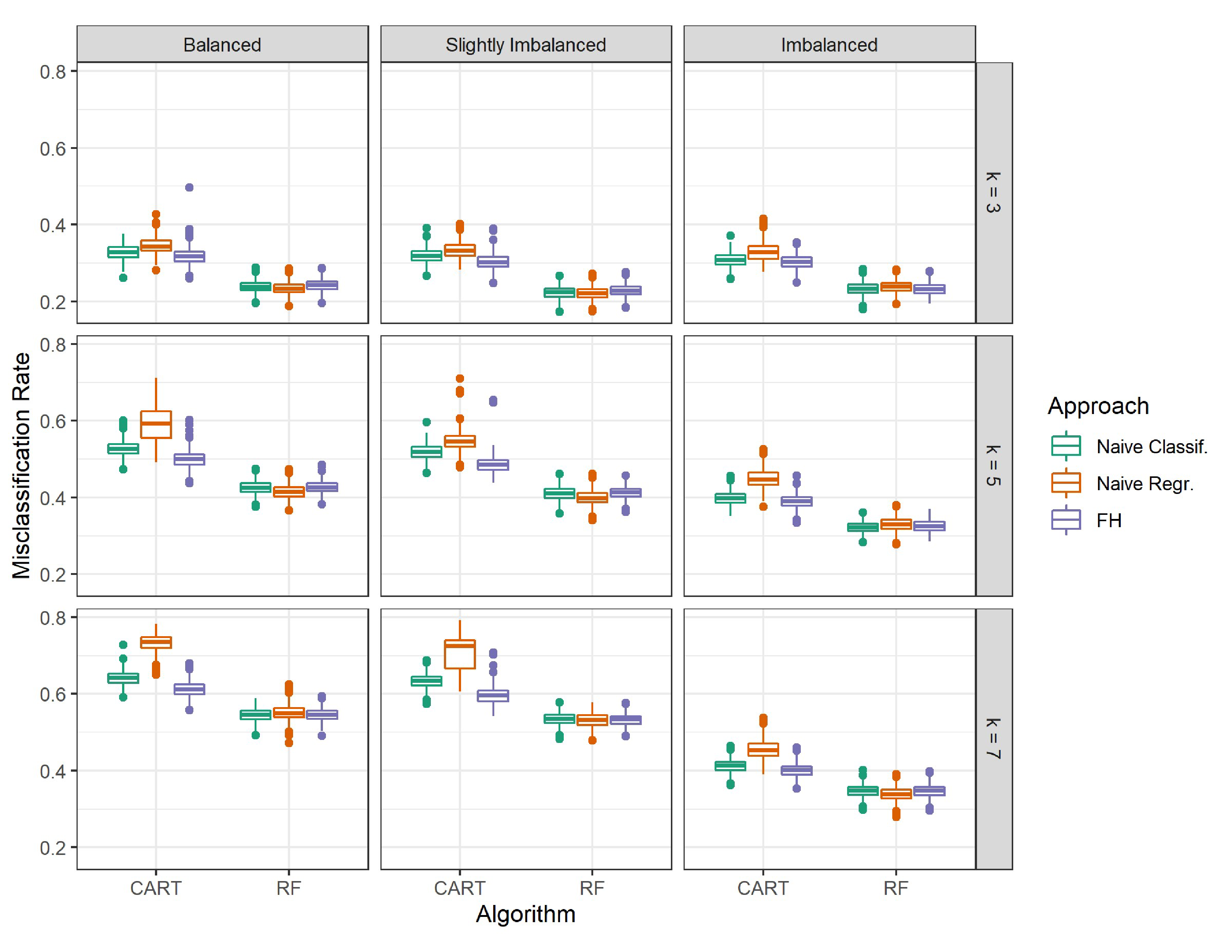Figure B.1: Distribution of misclassification rate of the six models across replications in each simulation condition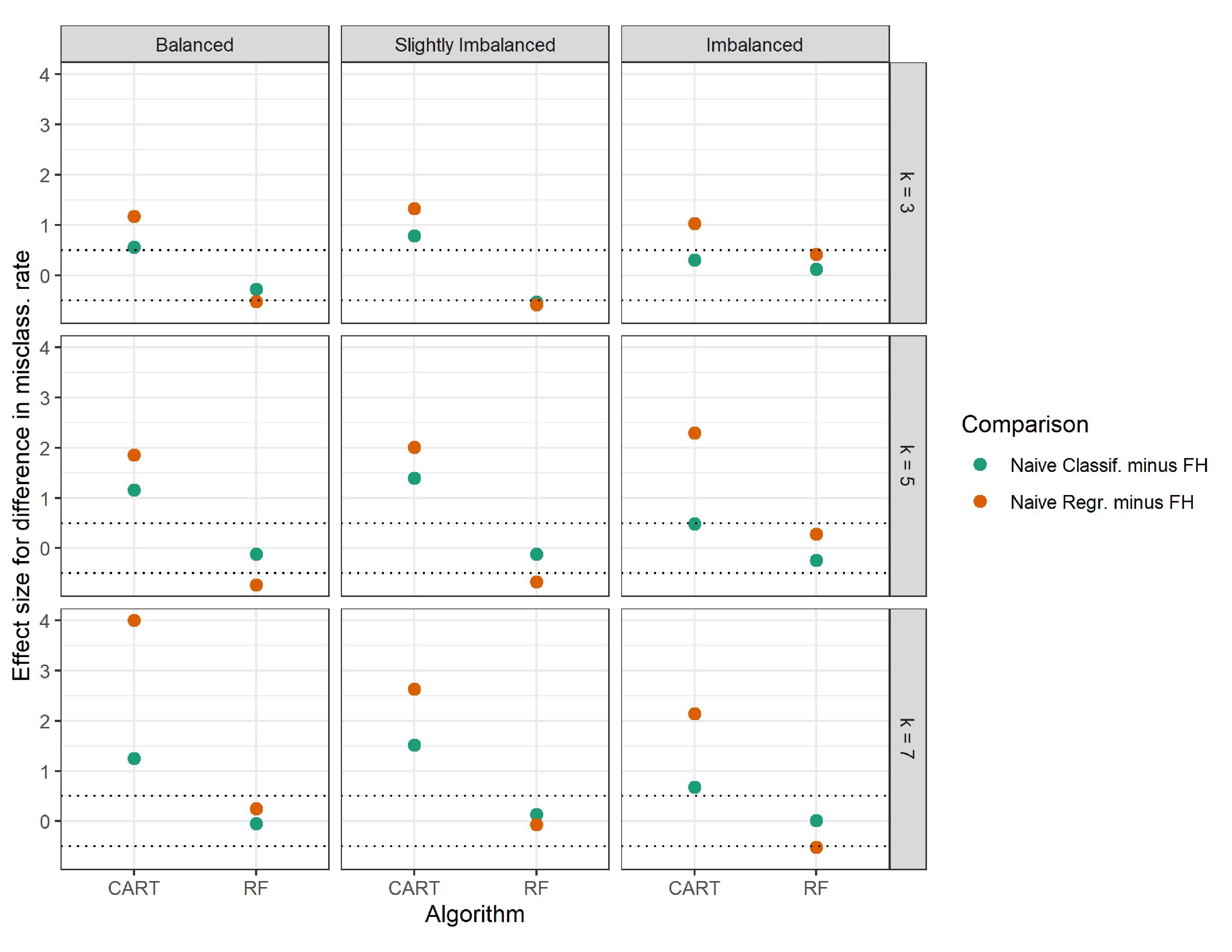Figure B.2: Effect sizes for the paired difference in misclassification rate between the FH approach and naïve approaches for each algorithm for each simulation condition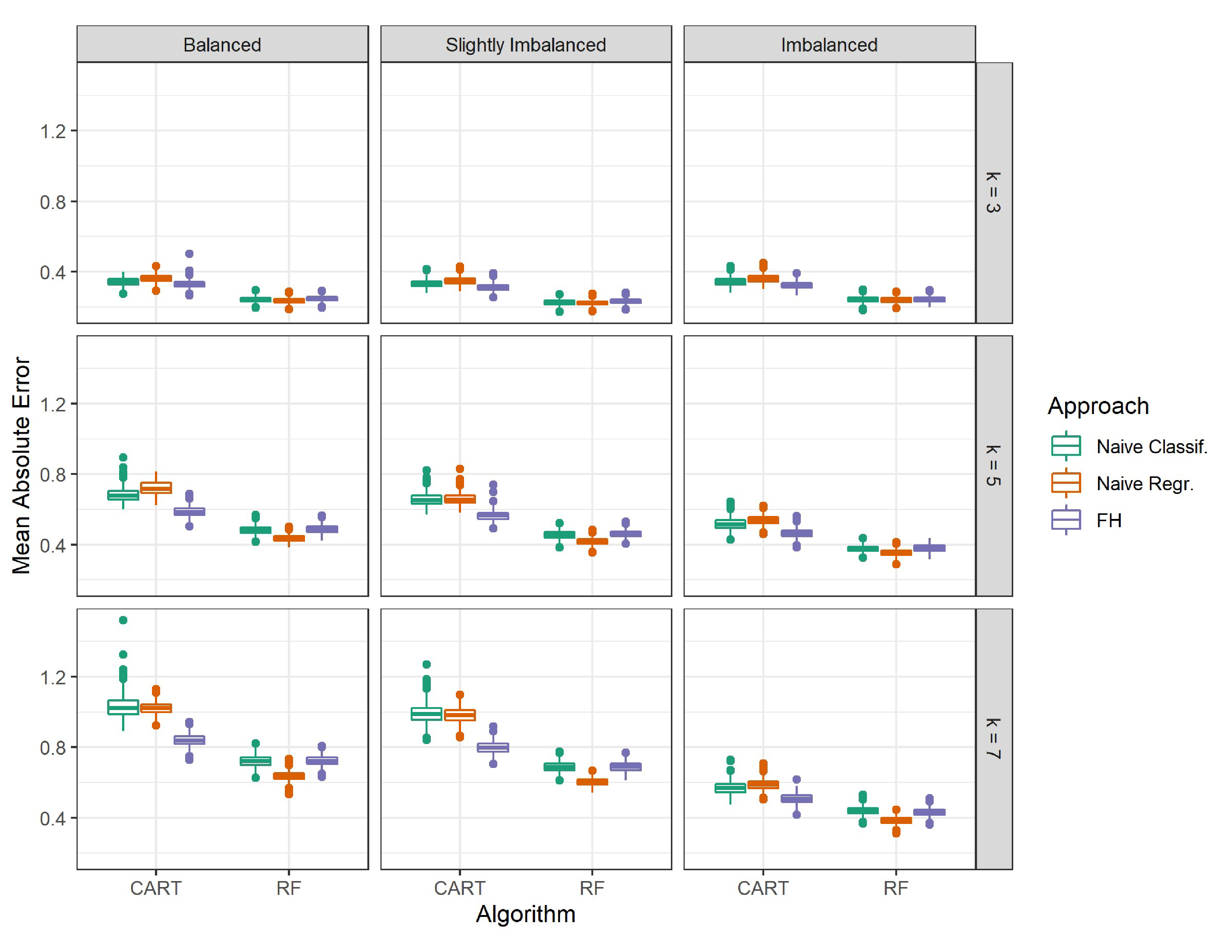Figure B.3: Distribution of mean absolute error of the six models across replications in each simulation condition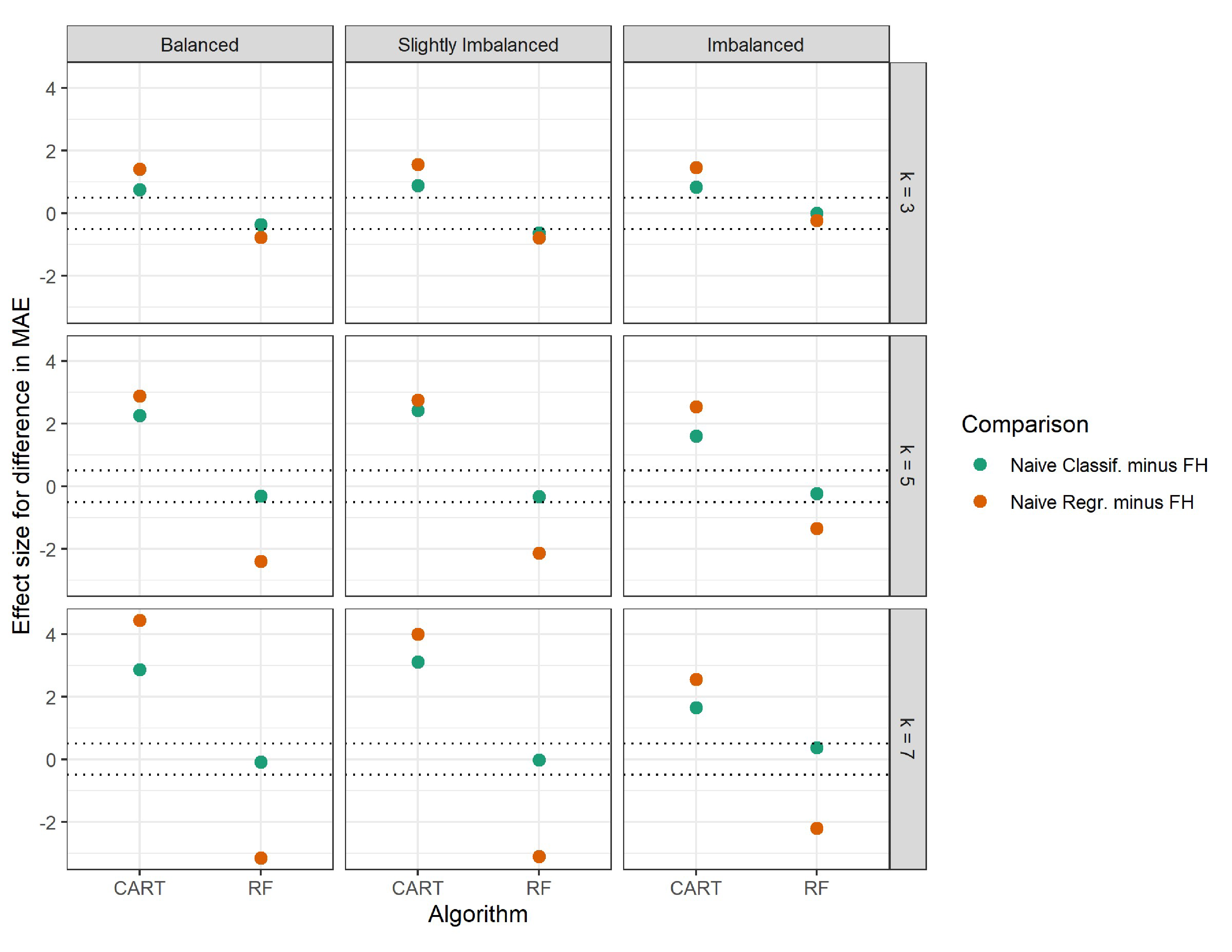Figure B.4: Effect sizes for the paired difference in mean absolute error between the FH approach and naïve approaches for each algorithm for each simulation condition